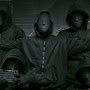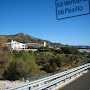# CLASS B SUBNET CALCULATIONS the Easy Waywelcome to my another video about subnetting I'm going to show you how to do a subnet calculations for a Class B networks it's a little bit different and I guess the major thing is that people may not know you just need to know where you need to start to do calculations so let's do a plus B sub no calculations so I pick the IP address right here it's a 172 that's 16 that 100 the 33 with a Class B subnet mask 255 255 dot 255 airing again okay now for a Class B networks you have to know where to start doing your calculations and I change the colors to show you this is the boundary for Class B networks right here which is the second octet so all your mass calculations will become the third and fourth octet okay that's very important so when you want to know how many networks and holes are available using a mask let's say with 252 that zero you know you have to start from here and here okay so let's find number of networks basically if you see my previous video of Class C subnets you have to know how many ones are in the mask and because this is the boundary we always stop at the boundary so we counting one two three four five six we have six ones that means 2 to the power of six will gives us number of networks which is 64 so let me write this down here number of networks 2 to the power of 6 which means six ones gives the number of networks using this subnet and each network is going to have so many hosts and to find out how many hosts is available account number of zeros 1 2 3 4 5 6 7 8 9 10 so it's basically 2 to the power of 10 which gives you 10 24 right here so we got 1024 available addresses but we cannot use 2 so we have to subtract 2 from it which gives us right let me type it equal 10 22 so this is how many hosts you can have using this mask on each Network so you can have 64 networks with 1022 hosts available so let's find what the subnet address is to find out what the subnet addresses you need to take the IP address and the mask and you have to do end operation between them so I took the last two outlets and I convert into binary so it's right here that 133 convert to binary is this and the mask it's this one right here I'm sorry this not to mask the mass is actually this one so let's see again let's copy that see at least this one there you go okay so this is your mask of 252 right here okay so you need to do end operation and to do end operation every time you have when you add numbers in binary it's not adding is just are doing operations so in the end operation to have a logical one you need to have two ones on so 0 & 1 will give you 0 1 and 1 gives you 1 1 1 1 0 1 0 and so on and our result is this right here so this is our answer when you do end operation so you only can have a 1 when there's two ones when you convert this to decimal it will give you this right here 100 0 so when you do your calculations by hand or by calculator during the test you cannot dyscalculia so you have to do it by hand anyway this is 100 0 and to find our broadcast address basically what you need to do if you take the mask and the boundary is right here let me draw the boundary the boundary is right here you can see at me you can get a thicker line so this is our boundary because this is the mask so when you draw the line right after the last one and you take your broad subnet address and you change these zeros into ones will give you a broadcast address which is this right here okay so I left these unchanged and I just change these zeros into ones and this is my broadcast address when you convert it into decimal it will give you like this okay so this IP address which is on Class B subnet lies within 100 that's 0 to 103 to 255 now to find a house range you basically need to subtract 1 which is a or you are you do plus 1 and minus 1 so there's going to be 101 and the broadcast will be 103 that 254 and this is my range right here there we go and this is how you do it I mean it's very simple you don't need to memorize any tables you just have to do everything in binary and just remember couple rules okay so let's go recap what we learned to do a Class B summary calculations you have to know where the boundary is for Class B which is the second end of the second act at beginning of the third one I change the colors to show you to find number of networks you do count how many ones are in the mask in this case is 6 2 to the power of 6 gives you 64 which is 64 networks to find my hosts you count how many zeros are in the mask in this case is 10 so it's 2 to the power of 10 gives you 1024 you subtract 2 because you cannot use the subnet address and broadcast address so it gives you a 1022 addresses 1022 to find your subnet address you have to do end operation between two numbers convert them to binary and the end operation result is here which convert to decimal gives you 100 0 and to find to find your broadcast address basically you take you have to know where your mask is and you copy the numbers within the mask and after the mask which is the zero so you have to convert them to ones when you convert them into the decimal it gives you 103 that 255 and of course this is our host range which you just add one here and you minus 1 here and this is your range I hope this video is informational and you learn something new I will try to do a class a submitting the reason thank you bye

#### 23 Comments

•Ezra G says:

Thank You!

•Whitehat Pro says:

I’m from 2018. This is brilliant. The concepts clicked 3 in the morning lmao.

•Nas KHan says:

very confusing.

•Nur Sakinah says:

Dammm!!Thank you so much for posting this video. Now i already know how to calculate subnet for Class B. Once again Thank you so much❤

•Miguel Jackson says:

This video is old but helped me a lot, thanks!

•StandardFresh says:

I've no-shit been looking at videos to explain this as well as you did; for about an hour. THANK YOU!

•Bryan Mendoza says:

Thanks sir for this very helpful video,, i hope to your next video is all about IPv6

•SGlocalGamer says:

Help I don't get the line part. Why is is position there?

•Alvin Aquino says:

In calculating the SUBNET ADDRESS, If you encounter two 0 is equal to 1?

•GalaxyTraveler says:

64 is the number of subnets? I thought you would normally minus 2 from that.

•Gagan Kaushik says:

Hats Off….

•Alex Lima says:

Saved my life! Thanks a lot for share knowledge.
You don't have to care about haters, you probably know "haters gonna hate"

•Mansoor Malik says:

how did you bring up broad cast address 103.255, please xplian

•LiberLotus says:

Hey thanks for the great vid. With a class B being 255.255.0.0, I'm wondering why you chose 252.0? What was that based on?

•Tevin Falkner says:

how did you get 252 in the third octet?

•Leia says:

helped a ton, thanks 🙂

•dvuemedia says:

@always and forever
64+32+4=100
32+1=33
thus 100.33

•Kimberly porladie says:

all this does is echos hard to understand

•always and forever says:

i dont know if this is a dumb question but…I am lost on how he got 0110 0100. 0010 0001 for 100. 33, can anyone explain?

•skote77 says:

thanks famm chyyyyl/10

•JOVA NNE says:

excellent video tutorial!! this is the most explanatory and simple way for subnetting!! thanks so much bro!!

•doghousemine1 says:

I have watched numerous videos on this subject.    You kept it simple thus very easy to understand.   Thanks for clarifying.    Nice Job.

•Eke Princewill says:

nice